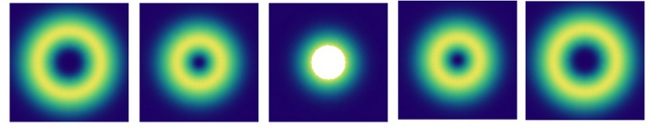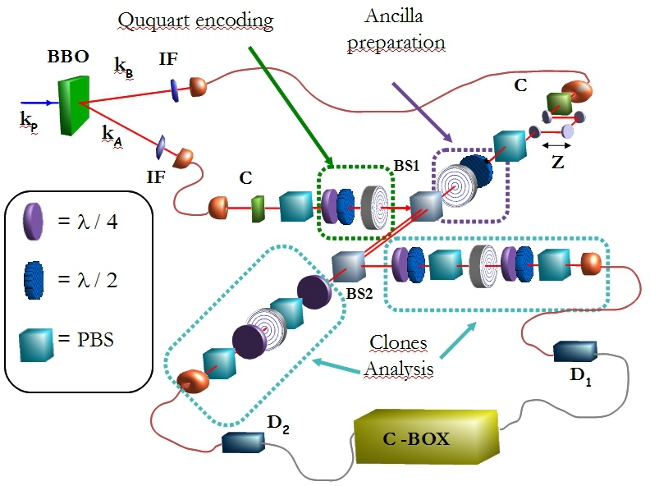The electromagnetic theory states that a beam of light carries both energy and momentum, in its linear and angular components. In 1992 it was demonstrated that specic light beams, solution of the Helmoltz equation in the paraxial approximation, characterized by a helicoidal phase profile along the z direction of propagation, carry a well defined value of angular momentum independently from the spin. Such component of AM is known as orbital angular momentum (OAM) and is related to the photon's transverse-mode spatial structure. Light beams that carry OAM, as for example Laguerre-Gauss modes (LG), are characterized by a phase factor eim' where m is the eigenvalue of the orbital angular momentum operator. Consistently with quantum theory, m can assume any positive or negative integer value, that physically refers to the number of twisting of the phase along z in clockwise (positive) or counter-clockwise (negative) orientation. Thus the orbital angular momentum, for some aspects similar to polarization, is in contrast defined in an infinite dimensional Hilbert space. For its features, in last decade the OAM has attracted much attention in different fields of science, from physics to biology. In particular it has been recognized as a new promising resource in quantum information implemented through quantum optics techniques, where it has been shown that the standard information encoding based on the two-dimensional quantum space of photon polarizations, or spin angular momentum, imposes significant limitations to the protocols that may be implemented. It has been demonstrated he orbital angular momentum of light could allow the implementation of a higher dimensional quantum space, known as qudit, encoded in a single photon [1,2]. Qudit-based quantum information protocols may also offer better theoretical performances than their qubit equivalents, while the combined use of different degrees of freedom of a photon, such as OAM and spin, enables the implementation of entirely new quantum tasks [3,4].In this context it is possible to include the research work in our group. Up to now in our experiments we have adopted a new optical device based on nematic liquid crystals, the q-plate, able to couple the photon spin to its orbital angular momentum thus opening a number of new possibilities in the field of light manipulation . We have manipulated qubits encoded in different bidimensional subspaces of OAM and implemented the first optimal quantum cloning of a OAM qubit . By exploiting both the polarization and the orbital angular momentum, we are now mainly focused on the study of higher dimensional quantum states and their adoption for quantum communication and information purposes. In this context we have efficiently manipulated ququart states, i.e. quantum states with dimensionality d=4, and implemented related quantum information protocols . We shown that such states could be successfully adopted to achieve a high stability and transmission rate in free-space propagation, and for the implementation of quantum cryptographic protocols such as quantum key distribution .